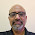## Sunday, July 8, 2018

### #PythaShastri two hands pi formula

#PythaShastri two hands pi formula is working. Here it is:
Well, (2n(2n-1) is left-hand and (3n(3n-1)(3n-2)) is the right hand. And it results in pi expansions as per Wolfram.

#### 1 comment:

1.This gives irrational numbers.
I am not sure of rational numbers and irrational numbers with pi.